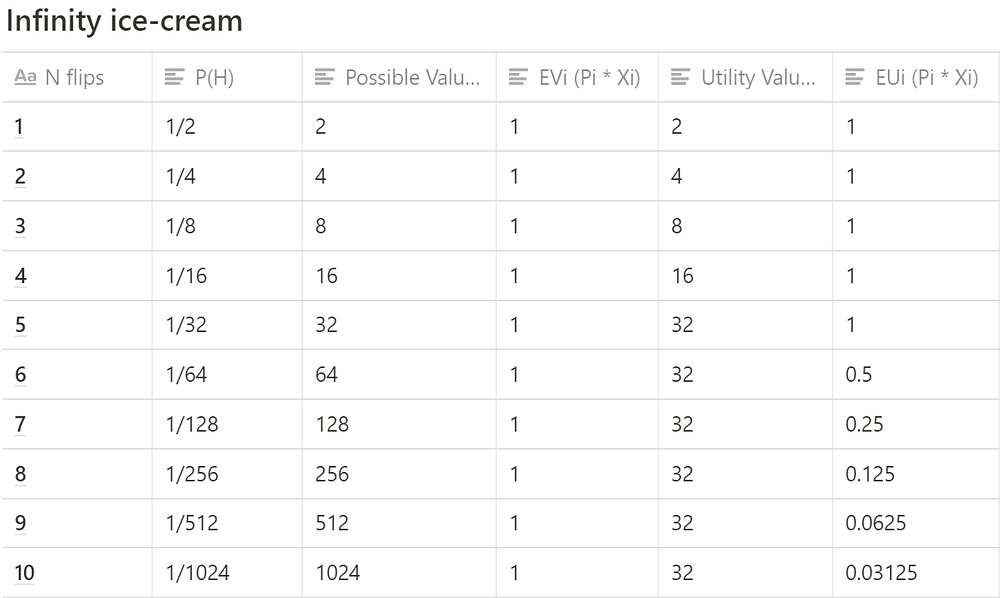Original article was published on Artificial Intelligence on MediumThe determination of the value of an item must not be based on the price, but rather on the utility it yields. There is no doubt that a gain of one thousand ducats is more significant to the pauper than to a rich man.” — Daniel Bernoulli

## Index

0. Dear Statistics Journal
1. Introduction to Probability
2. The Monty Hall Problem
3. Gambler’s Fallacy

Before we get to the good stuff, let’s knock out a few useful concepts:
. Random Variables
. Expected Value
. Expected Utility

# Random Variables

A random variable is a function that maps the outcome of a random process to a real number.

E.g., say we have the random process of flipping a fair coin. X is essentially a function that maps values of the sample space ({Heads, Tails}) to a real number. In a sense, we are quantifying the state space of outcomes. As seen in Fig 1, we assign the values Heads=0 & Tails=1 and X is our random variable for this particular process.

# Expected Value

Expected value is the average value of a random variable over a large number of experiments